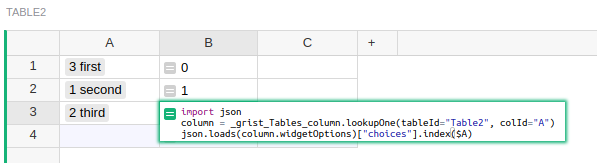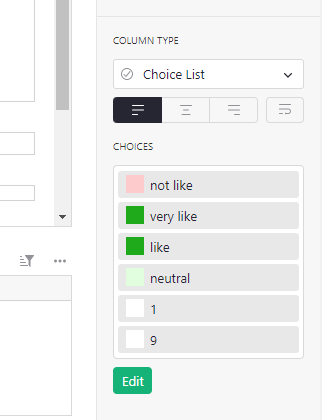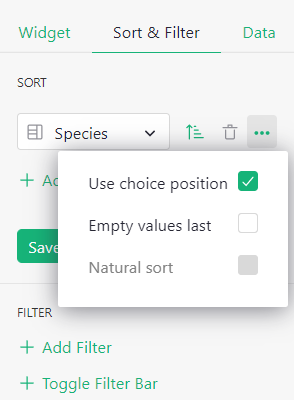# Feature Request - Column Sort by Label Order

When sorting a Choice column, it’d be great if we can sort by the label order (assigned by the user), not just alphabetically.

1 Like

Ooh, makes sense! Thanks for sharing the idea.

You can do this by hacking into Grist with an extra formula like this:

``````import json
column = _grist_Tables_column.lookupOne(tableId="Table2", colId="A")
``````

This gives the position of `\$A` within the choices of the column `A` in `Table2`, so you can sort by that.

2 Likes

Thanks, but somehow I can’t make it work…

Here’s a demo: GristI think I’m doing exactly that, but it’s still not sorting… by the way, can you please make the formula “box” bigger?1. The formula I gave will only work for Choice columns. A different formula is needed for Choice List columns. What do you need?
2. The formula needs to be in a separate column from the Choice column. It returns a number which is the position of the choice. That’s the column to sort by. You’ve put the formula in the same column which means something different (Intro to formulas - Grist Help Center)

This is why I requested a feature that a layperson can use. Not a developer here…Update: @school

You can now sort a Choice column by the label order in the choice editor. When sorting a Choice column, click on the three dots to open a menu of additional features. “Use choice position” sorts by label order.2 Likes